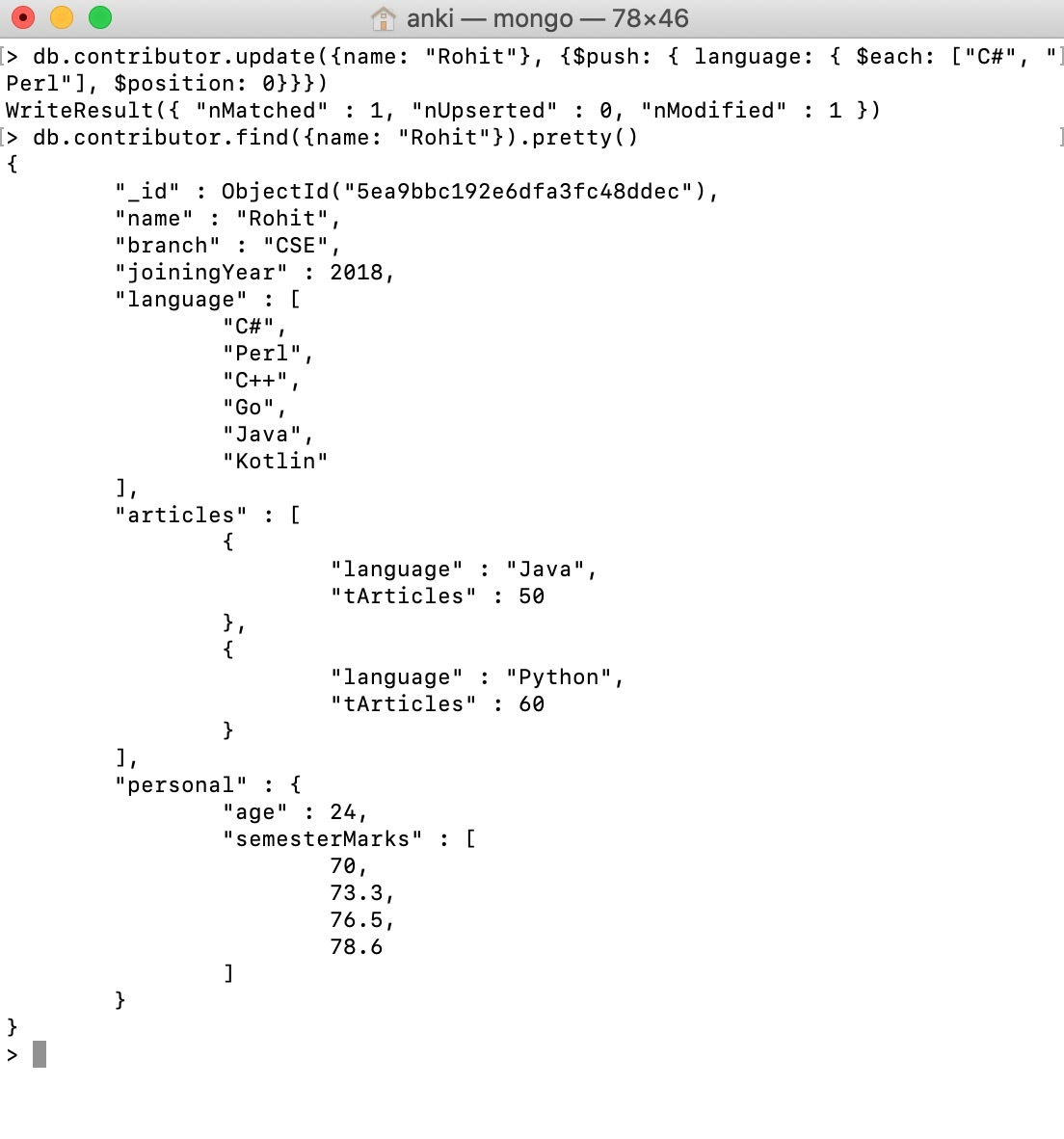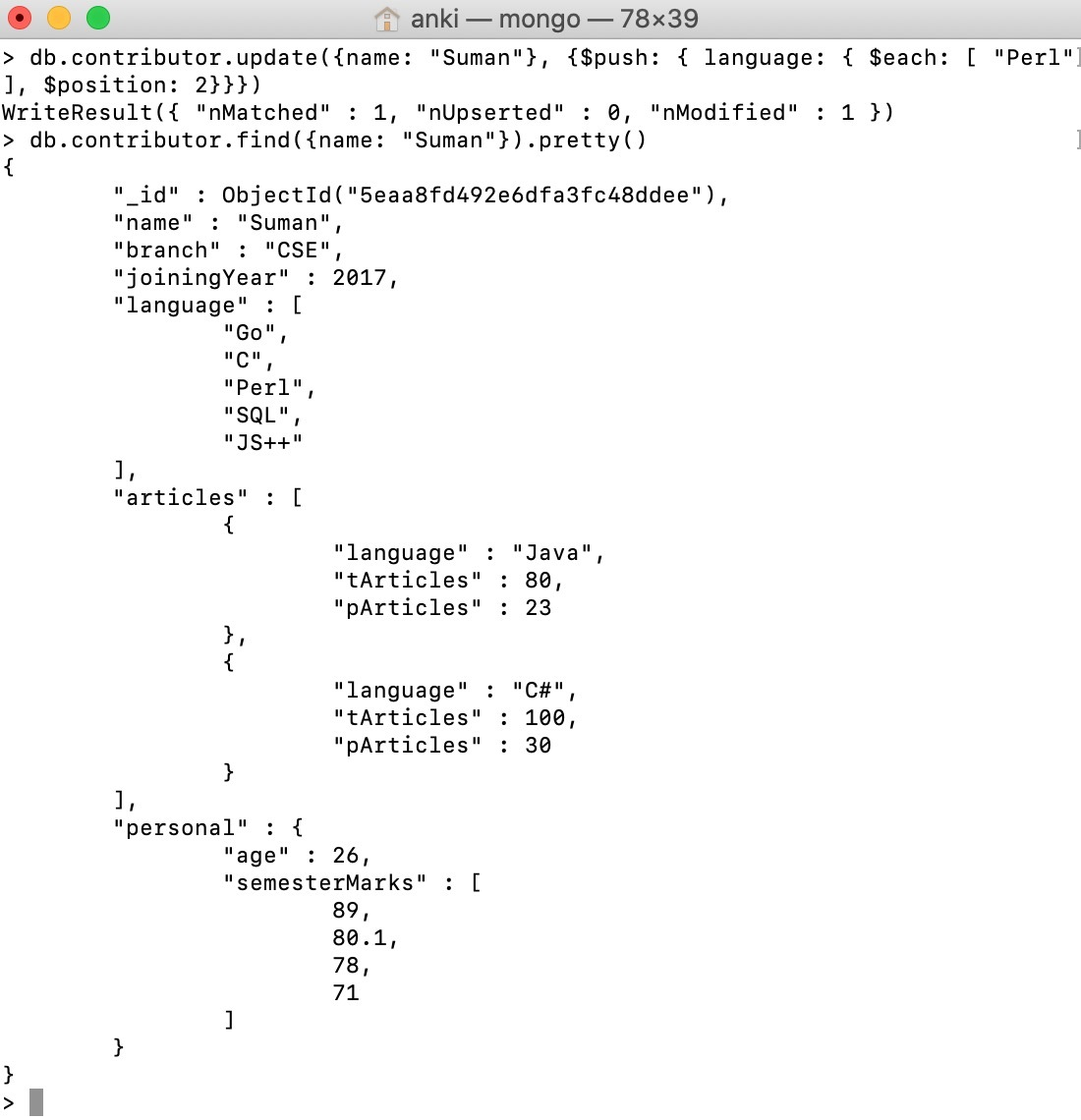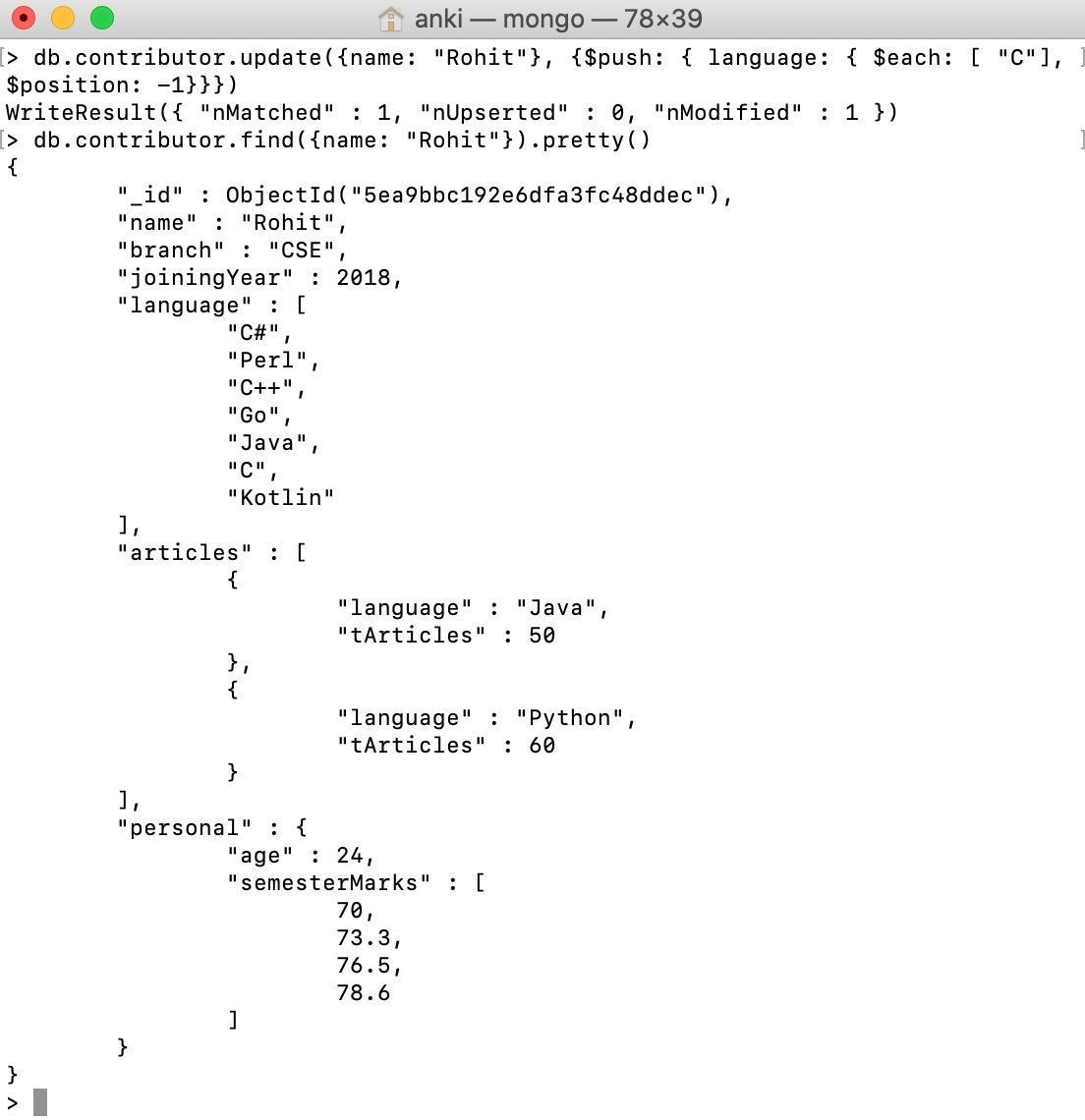GFG App
Open AppBrowser
Continue

MongoDB provides different types of array update operators to update the values of the array fields in the documents and `\$position ` modifier is one of them. This modifier is used to specify the location in the array at which the `\$push` operator inserts items. Without \$position modifier \$push operator insert items at the end of the array.

Syntax:

```{
\$push: {
<field>: {
\$each: [ <value1>, <value2>, ... ],
\$position: <number>
}
}
}```

Here, <number> indicates the position of the item in the array according to the zero-based index.

• If the value of <number> (non-negative number corresponds to the array which is starting from the beginning of the array) is greater or equal to the length of the array, then this modifier does not work and the` \$push` operation adds items to the end of the array.
• Starting from MongoDB version 3.6, `\$position` modifier accepts the negative index value. When the value of <number> is negative, then the \$position operation counts the item from the last of the array, but does not include the last element. For example, if the value of \$position modifier is -1, then it indicates the position just before the last item in the array and if you specify multiple items in the \$each array, then the last added item is in the specified position from the end. If the value of <number> is greater than or equal to the length of the array, then the \$push operation adds items to the beginning of the array.
• `\$position` modifier must appear with \$each modifier in the \$push operator. If you use the \$position modifier without \$each modifier, then you will get an error.

In the following examples, we are working with:

Database: GeeksforGeeks
Collection: contributor
Document: two documents that contain the details of the contributor in the form of field-value pairs.

## Adding items at the start of the array:

In this example, we are adding items, i.e., [“C#”, “Perl”] in the beginning(i.e., position 0 )of the language field.

 `db.contributor.update({name: ``"Rohit"``},  ` `                      ``{\$push: { language: { \$each: [``"C#"``, ``"Perl"``], ` `                        ``\$position: ``0``}}})`## Adding items to the middle of the array:

In this example, we are adding items, i.e., [“Perl”] in the middle(i.e., position 2 )of the language field.

 `db.contributor.update({name: ``"Suman"``},  ` `                      ``{\$push: { language: { \$each: [ ``"Perl"``],  ` `                        ``\$position: ``2``}}})`## Using a negative index to add items to the array:

In this example, we are adding items, i.e., [“C”] just before the last item in the language field.

 `db.contributor.update({name: ``"Rohit"``}, ` `                      ``{\$push: { language: { \$each: [ ``"C"``],  ` `                        ``\$position: ``-``1``}}})`My Personal Notes arrow_drop_up